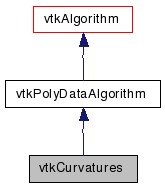# vtkCurvatures Class Reference

#include <vtkCurvatures.h>

Inheritance diagram for vtkCurvatures:[legend]
Collaboration diagram for vtkCurvatures:[legend]

## Detailed Description

compute curvatures (Gauss and mean) of a Polydata object

vtkCurvatures takes a polydata input and computes the curvature of the mesh at each point. Four possible methods of computation are available :

Gauss Curvature discrete Gauss curvature (K) computation,The contribution of every facet is for the moment weighted byThe units of Gaussian Curvature areMean CurvatureNB: dihedral_angle is the ORIENTED angle between -PI and PI, this means that the surface is assumed to be orientable the computation creates the orientation The units of Mean Curvature are [1/m]

Maximum () and Minimum () Principal CurvaturesExcepting spherical and planar surfaces which have equal principal curvatures, the curvature at a point on a surface varies with the direction one "sets off" from the point. For all directions, the curvature will pass through two extrema: a minimum () and a maximum () which occur at mutually orthogonal directions to each other.

NB. The sign of the Gauss curvature is a geometric ivariant, it should be +ve when the surface looks like a sphere, -ve when it looks like a saddle, however, the sign of the Mean curvature is not, it depends on the convention for normals - This code assumes that normals point outwards (ie from the surface of a sphere outwards). If a given mesh produces curvatures of opposite senses then the flag InvertMeanCurvature can be set and the Curvature reported by the Mean calculation will be inverted.

Thanks:
Philip Batchelor philipp.batchelor@kcl.ac.uk for creating and contributing the class and Andrew Maclean a.maclean@acfr.usyd.edu.au for cleanups and fixes. Thanks also to Goodwin Lawlor for contributing patch to calculate principal curvatures
Tests:
vtkCurvatures (Tests)

Definition at line 75 of file vtkCurvatures.h.

virtual void SetInvertMeanCurvature (int)
virtual int GetInvertMeanCurvature ()
virtual void InvertMeanCurvatureOn ()
virtual void InvertMeanCurvatureOff ()
vtkCurvatures ()

## Public Types

typedef vtkPolyDataAlgorithm Superclass

## Public Member Functions

virtual const char * GetClassName ()
virtual int IsA (const char *type)
void PrintSelf (ostream &os, vtkIndent indent)
virtual void SetCurvatureType (int)
virtual int GetCurvatureType ()
void SetCurvatureTypeToGaussian ()
void SetCurvatureTypeToMean ()
void SetCurvatureTypeToMaximum ()
void SetCurvatureTypeToMinimum ()

## Static Public Member Functions

static int IsTypeOf (const char *type)
static vtkCurvaturesSafeDownCast (vtkObject *o)
static vtkCurvaturesNew ()

## Protected Member Functions

int RequestData (vtkInformation *, vtkInformationVector **, vtkInformationVector *)
void GetGaussCurvature (vtkPolyData *output)
void GetMeanCurvature (vtkPolyData *output)
void GetMaximumCurvature (vtkPolyData *input, vtkPolyData *output)
void GetMinimumCurvature (vtkPolyData *input, vtkPolyData *output)

## Protected Attributes

int CurvatureType
int InvertMeanCurvature

## Member Typedef Documentation

Reimplemented from vtkPolyDataAlgorithm.

Definition at line 78 of file vtkCurvatures.h.

## Constructor & Destructor Documentation

 vtkCurvatures::vtkCurvatures ( )  [protected]

Set/Get the flag which inverts the mean curvature calculation for meshes with inward pointing normals (default false)

## Member Function Documentation

 virtual const char* vtkCurvatures::GetClassName ( )  [virtual]

Reimplemented from vtkPolyDataAlgorithm.

 static int vtkCurvatures::IsTypeOf ( const char * name )  [static]

Return 1 if this class type is the same type of (or a subclass of) the named class. Returns 0 otherwise. This method works in combination with vtkTypeMacro found in vtkSetGet.h.

Reimplemented from vtkPolyDataAlgorithm.

 virtual int vtkCurvatures::IsA ( const char * name )  [virtual]

Return 1 if this class is the same type of (or a subclass of) the named class. Returns 0 otherwise. This method works in combination with vtkTypeMacro found in vtkSetGet.h.

Reimplemented from vtkPolyDataAlgorithm.

 static vtkCurvatures* vtkCurvatures::SafeDownCast ( vtkObject * o )  [static]

Reimplemented from vtkPolyDataAlgorithm.

 void vtkCurvatures::PrintSelf ( ostream & os, vtkIndent indent )  [virtual]

Methods invoked by print to print information about the object including superclasses. Typically not called by the user (use Print() instead) but used in the hierarchical print process to combine the output of several classes.

Reimplemented from vtkPolyDataAlgorithm.

 static vtkCurvatures* vtkCurvatures::New ( )  [static]

Construct with curvature type set to Gauss

Reimplemented from vtkPolyDataAlgorithm.

 virtual void vtkCurvatures::SetCurvatureType ( int )  [virtual]

Set/Get Curvature type VTK_CURVATURE_GAUSS: Gaussian curvature, stored as DataArray "Gauss_Curvature" VTK_CURVATURE_MEAN : Mean curvature, stored as DataArray "Mean_Curvature"

 virtual int vtkCurvatures::GetCurvatureType ( )  [virtual]

Set/Get Curvature type VTK_CURVATURE_GAUSS: Gaussian curvature, stored as DataArray "Gauss_Curvature" VTK_CURVATURE_MEAN : Mean curvature, stored as DataArray "Mean_Curvature"

 void vtkCurvatures::SetCurvatureTypeToGaussian ( )  [inline]

Set/Get Curvature type VTK_CURVATURE_GAUSS: Gaussian curvature, stored as DataArray "Gauss_Curvature" VTK_CURVATURE_MEAN : Mean curvature, stored as DataArray "Mean_Curvature"

Definition at line 90 of file vtkCurvatures.h.

 void vtkCurvatures::SetCurvatureTypeToMean ( )  [inline]

Set/Get Curvature type VTK_CURVATURE_GAUSS: Gaussian curvature, stored as DataArray "Gauss_Curvature" VTK_CURVATURE_MEAN : Mean curvature, stored as DataArray "Mean_Curvature"

Definition at line 92 of file vtkCurvatures.h.

 void vtkCurvatures::SetCurvatureTypeToMaximum ( )  [inline]

Set/Get Curvature type VTK_CURVATURE_GAUSS: Gaussian curvature, stored as DataArray "Gauss_Curvature" VTK_CURVATURE_MEAN : Mean curvature, stored as DataArray "Mean_Curvature"

Definition at line 94 of file vtkCurvatures.h.

 void vtkCurvatures::SetCurvatureTypeToMinimum ( )  [inline]

Set/Get Curvature type VTK_CURVATURE_GAUSS: Gaussian curvature, stored as DataArray "Gauss_Curvature" VTK_CURVATURE_MEAN : Mean curvature, stored as DataArray "Mean_Curvature"

Definition at line 96 of file vtkCurvatures.h.

 virtual void vtkCurvatures::SetInvertMeanCurvature ( int )  [virtual]

Set/Get the flag which inverts the mean curvature calculation for meshes with inward pointing normals (default false)

 virtual int vtkCurvatures::GetInvertMeanCurvature ( )  [virtual]

Set/Get the flag which inverts the mean curvature calculation for meshes with inward pointing normals (default false)

 virtual void vtkCurvatures::InvertMeanCurvatureOn ( )  [virtual]

Set/Get the flag which inverts the mean curvature calculation for meshes with inward pointing normals (default false)

 virtual void vtkCurvatures::InvertMeanCurvatureOff ( )  [virtual]

Set/Get the flag which inverts the mean curvature calculation for meshes with inward pointing normals (default false)

 int vtkCurvatures::RequestData ( vtkInformation * request, vtkInformationVector ** inputVector, vtkInformationVector * outputVector )  [protected, virtual]

This is called by the superclass. This is the method you should override.

Reimplemented from vtkPolyDataAlgorithm.

 void vtkCurvatures::GetGaussCurvature ( vtkPolyData * output )  [protected]

discrete Gauss curvature (K) computation, cf http://www-ipg.umds.ac.uk/p.batchelor/curvatures/curvatures.html

 void vtkCurvatures::GetMeanCurvature ( vtkPolyData * output )  [protected]

 void vtkCurvatures::GetMaximumCurvature ( vtkPolyData * input, vtkPolyData * output )  [protected]

Maximum principal curvaturevoid vtkCurvatures::GetMinimumCurvature ( vtkPolyData * input, vtkPolyData * output )  [protected]

Minimum principal curvature## Member Data Documentation

 int vtkCurvatures::CurvatureType [protected]

Definition at line 129 of file vtkCurvatures.h.

 int vtkCurvatures::InvertMeanCurvature [protected]

Definition at line 130 of file vtkCurvatures.h.

The documentation for this class was generated from the following file:

Generated on Wed Aug 24 11:32:45 2011 for VTK by1.5.6